Work, Energy, and Power - Lesson 2 - The Work-Energy Relationship

# Application and Practice Questions

Lesson 2 has thus far focused on how to analyze motion situations using the work and energy relationship. The relationship could be summarized by the following statements:

 There is a relationship between work and mechanical energy change. Whenever work is done upon an object by an external or nonconservative force, there will be a change in the total mechanical energy of the object. If only internal forces are doing work (no work done by external forces), there is no change in total mechanical energy; the total mechanical energy is said to be "conserved." The quantitative relationship between work and the two forms of mechanical energy is expressed by the following equation: KEi + PEi + Wext = KEf + PEf

Now an effort will be made to apply this relationship to a variety of motion scenarios in order to test our understanding.

## .ad3Container { margin-top: 10px; } .adslot_3 { width: 320px!important; height: 100px!important; } @media (min-width:992px) { .adslot_3 { width: 728px; height: 90px; } } @media (max-width:767px) { .ad3Container { display: block; left: 0; position: absolute; top: -740px; width: 100%; } .adslot_3 { display: block !important; height: 100px!important; margin-left: auto; margin-right: auto; text-align: center; width: 320px!important; } .navbar .container { padding-top: 80px; } #bannerHolder > img { height: 75px; } } (adsbygoogle = window.adsbygoogle || []).push({});

Use your understanding of the work-energy theorem to answer the following questions. Then click the button to view the answers.

1. Consider the falling and rolling motion of the ball in the following two resistance-free situations. In one situation, the ball falls off the top of the platform to the floor. In the other situation, the ball rolls from the top of the platform along the staircase-like pathway to the floor. For each situation, indicate what types of forces are doing work upon the ball. Indicate whether the energy of the ball is conserved and explain why. Finally, fill in the blanks for the 2-kg ball.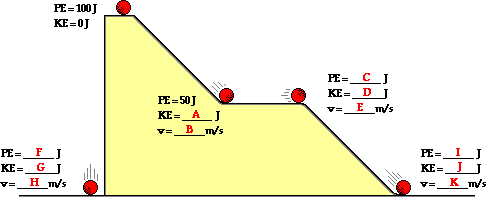2. If frictional forces and air resistance were acting upon the falling ball in #1 would the kinetic energy of the ball just prior to striking the ground be more, less, or equal to the value predicted in #1?

Use the following diagram to answer questions #3 - #5. Neglect the effect of resistance forces.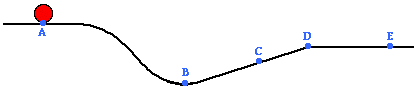3. As the object moves from point A to point D across the surface, the sum of its gravitational potential and kinetic energies ____.

 a. decreases, only b. decreases and then increases c. increases and then decreases d. remains the same

4. The object will have a minimum gravitational potential energy at point ____.

 a. A b. B c. C d. D e. E

5. The object's kinetic energy at point C is less than its kinetic energy at point ____.

 a. A only b. A, D, and E c. B only d. D and E

6. Many drivers' education books provide tables that relate a car's braking distance to the speed of the car (see table below). Utilize what you have learned about the stopping distance-velocity relationship to complete the table.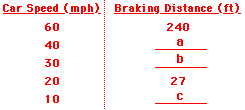7. Some driver's license exams have the following question.

A car moving 50 km/hr skids 15 meters with locked brakes. How far will the car skid with locked brakes if it is moving at 150 km/hr?

8. Two baseballs are fired into a pile of hay. If one has twice the speed of the other, how much farther does the faster baseball penetrate? (Assume that the force of the haystack on the baseballs is constant).

9. Use the law of conservation of energy (assume no friction) to fill in the blanks at the various marked positions for a 1000-kg roller coaster car.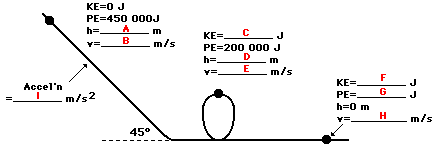10. If the angle of the initial drop in the roller coaster diagram above were 60 degrees (and all other factors were kept constant), would the speed at the bottom of the hill be any different? Explain.

11. Determine American ski jumper Lee Ben Fardest's (a mass of approximately 50 kg) speed at locations B, C, D and E.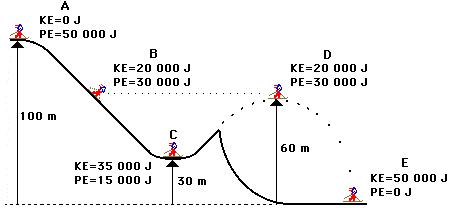12. An object which weighs 10 N is dropped from rest from a height of 4 meters above the ground. When it has free-fallen 1 meter its total mechanical energy with respect to the ground is ____.

 a. 2.5 J b. 10 J c. 30 J d. 40 J

13. During a certain time interval, a 20-N object free-falls 10 meters. The object gains _____ Joules of kinetic energy during this interval.

 a. 10 b. 20 c. 200 d. 2000

14. A rope is attached to a 50.0-kg crate to pull it up a frictionless incline at constant speed to a height of 3-meters. A diagram of the situation and a free-body diagram are shown below. Note that the force of gravity has two components (parallel and perpendicular component); the parallel component balances the applied force and the perpendicular component balances the normal force.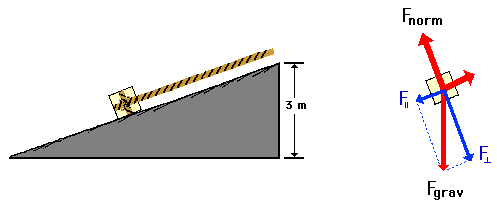Of the forces acting upon the crate, which one(s) do work upon it?

Based upon the types of forces acting upon the system and their classification as internal or external forces, is energy conserved? Explain.

Calculate the amount of work done upon the crate.

### We Would Like to Suggest ...Sometimes it isn't enough to just read about it. You have to interact with it! And that's exactly what you do when you use one of The Physics Classroom's Interactives. We would like to suggest that you combine the reading of this page with the use of our Roller Coaster Model Interactive, our Mass on a Spring Interactive, and/or our Chart That Motion Interactive. These three Interactives can be found in the Physics Interactive section of our website and provide an interactive opportunity to explore the work-energy relationship.

Next Section: